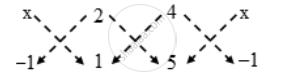Share

# For what value of x will the points (x, –1), (2, 1) and (4, 5) lie on a line ? - CBSE Class 10 - Mathematics

#### Question

For what value of x will the points (x, –1), (2, 1) and (4, 5) lie on a line ?

#### Solution

Given points will be collinear if the area of the triangle formed by them is zero.

∴ Area of the triangle = 0|{x × 1 + 2 × 5 + 4 × (–1)} – {(2 × (–1) + 4 × 1 + x × 5}| = 0

⇒ (x + 10 – 4) – (–2 + 4 + 5x) = 0

⇒ (x + 6) – (5x + 2) = 0

⇒ – 4x + 4 = 0

⇒ x = 1

Hence, the given points lies on a line, if x = 1.

Is there an error in this question or solution?

#### Video TutorialsVIEW ALL 

Solution For what value of x will the points (x, –1), (2, 1) and (4, 5) lie on a line ? Concept: Area of a Triangle.
S